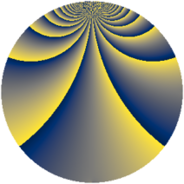# Properties

 Label 361.2.kLevel $361$ Weight $2$ Character orbit 361.k Rep. character $\chi_{361}(4,\cdot)$ Character field $\Q(\zeta_{171})$ Dimension $3348$ Newform subspaces $1$ Sturm bound $63$ Trace bound $0$

# Related objects

## Defining parameters

 Level: $$N$$ $$=$$ $$361 = 19^{2}$$ Weight: $$k$$ $$=$$ $$2$$ Character orbit: $$[\chi]$$ $$=$$ 361.k (of order $$171$$ and degree $$108$$) Character conductor: $$\operatorname{cond}(\chi)$$ $$=$$ $$361$$ Character field: $$\Q(\zeta_{171})$$ Newform subspaces: $$1$$ Sturm bound: $$63$$ Trace bound: $$0$$

## Dimensions

The following table gives the dimensions of various subspaces of $$M_{2}(361, [\chi])$$.

Total New Old
Modular forms 3564 3564 0
Cusp forms 3348 3348 0
Eisenstein series 216 216 0

## Trace form

 $$3348 q - 108 q^{2} - 111 q^{3} - 114 q^{4} - 108 q^{5} - 117 q^{6} - 111 q^{7} - 120 q^{8} - 117 q^{9} + O(q^{10})$$ $$3348 q - 108 q^{2} - 111 q^{3} - 114 q^{4} - 108 q^{5} - 117 q^{6} - 111 q^{7} - 120 q^{8} - 117 q^{9} - 123 q^{10} - 111 q^{11} - 117 q^{12} - 111 q^{13} - 111 q^{14} - 117 q^{15} - 96 q^{16} - 3 q^{17} - 108 q^{18} - 102 q^{19} - 132 q^{20} - 114 q^{21} - 120 q^{23} - 129 q^{24} - 114 q^{25} - 105 q^{26} - 63 q^{27} - 120 q^{28} - 111 q^{29} - 90 q^{30} - 123 q^{31} - 123 q^{32} + 9 q^{33} - 114 q^{34} - 120 q^{35} - 90 q^{36} - 114 q^{37} - 42 q^{38} - 126 q^{39} - 114 q^{40} - 135 q^{41} - 111 q^{42} + 3 q^{43} - 123 q^{44} - 84 q^{45} - 96 q^{46} - 111 q^{47} + 516 q^{48} - 24 q^{49} - 99 q^{50} - 117 q^{51} - 129 q^{52} - 111 q^{53} + 483 q^{54} - 132 q^{55} - 108 q^{56} - 138 q^{57} - 174 q^{58} - 126 q^{59} - 108 q^{60} - 102 q^{61} - 102 q^{62} - 126 q^{63} + 6 q^{64} - 102 q^{65} - 105 q^{66} - 84 q^{67} - 12 q^{68} + 411 q^{69} + 351 q^{70} - 108 q^{71} - 102 q^{72} - 102 q^{73} - 15 q^{74} - 144 q^{75} - 150 q^{76} - 162 q^{77} - 129 q^{78} - 75 q^{79} - 117 q^{80} - 120 q^{81} - 60 q^{82} - 90 q^{83} - 117 q^{84} - 114 q^{85} + 888 q^{86} - 63 q^{87} - 123 q^{88} - 102 q^{89} + 894 q^{90} - 15 q^{91} - 156 q^{92} - 123 q^{93} - 132 q^{94} + 75 q^{95} - 276 q^{96} - 132 q^{97} - 105 q^{98} - 123 q^{99} + O(q^{100})$$

## Decomposition of $$S_{2}^{\mathrm{new}}(361, [\chi])$$ into newform subspaces

Label Dim $A$ Field CM Traces $q$-expansion
$a_{2}$ $a_{3}$ $a_{5}$ $a_{7}$
361.2.k.a $3348$ $2.883$ None $$-108$$ $$-111$$ $$-108$$ $$-111$$